Courses

# a, b, and c are integers and a < b < c. S is the set of all integers from a to b, inclusive. Q is the set of all integers from b to c, inclusive. The median of set S is (3/4)b. The median of set Q is (7/8)c. If R is the set of all integers from a to c, inclusive, what fraction of c is the median of set R?a)3/8b)1/2c)11/16d)5/7e)3/4Correct answer is option 'C'. Can you explain this answer? Related Test: GMAT Full Mock Test- 1

## GMAT QuestionDEVASYA SACHAN Apr 11, 2020
Since S contains only consecutive integers, its median is the average of the extreme
values a and b. We also know that the median of S is 3/4b. We can set up and simplify
the following equation: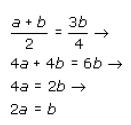Since set Q contains only consecutive integers, its median is also the average of the
extreme values, in this case b and c. We also know that the median of Q is 7/8c . We can
set up and simplify the following equation: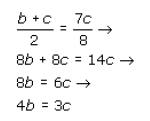We can find the ratio of a to c as follows:
Taking the first equation
2a =b→8a = 4b
and the second equation, 4b = 3c
and setting them equal to each other, yields the following: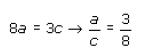Since set R contains only consecutive integers, its median is the average of the extreme
values a and c: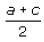. We can use the ratio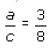to substitute 3c/8 for a: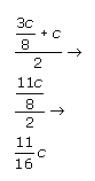Thus the median of set R is 11/16c. The correct answer is C.

This discussion on a, b, and c are integers and a < b < c. S is the set of all integers from a to b, inclusive. Q is the set of all integers from b to c, inclusive. The median of set S is (3/4)b. The median of set Q is (7/8)c. If R is the set of all integers from a to c, inclusive, what fraction of c is the median of set R?a)3/8b)1/2c)11/16d)5/7e)3/4Correct answer is option 'C'. Can you explain this answer? is done on EduRev Study Group by GMAT Students. The Questions and Answers of a, b, and c are integers and a < b < c. S is the set of all integers from a to b, inclusive. Q is the set of all integers from b to c, inclusive. The median of set S is (3/4)b. The median of set Q is (7/8)c. If R is the set of all integers from a to c, inclusive, what fraction of c is the median of set R?a)3/8b)1/2c)11/16d)5/7e)3/4Correct answer is option 'C'. Can you explain this answer? are solved by group of students and teacher of GMAT, which is also the largest student community of GMAT. If the answer is not available please wait for a while and a community member will probably answer this soon. You can study other questions, MCQs, videos and tests for GMAT on EduRev and even discuss your questions like a, b, and c are integers and a < b < c. S is the set of all integers from a to b, inclusive. Q is the set of all integers from b to c, inclusive. The median of set S is (3/4)b. The median of set Q is (7/8)c. If R is the set of all integers from a to c, inclusive, what fraction of c is the median of set R?a)3/8b)1/2c)11/16d)5/7e)3/4Correct answer is option 'C'. Can you explain this answer? over here on EduRev! Apart from being the largest GMAT community, EduRev has the largest solved Question bank for GMAT.